Tamilnadu State Board New Syllabus Samacheer Kalvi 11th Maths Guide Pdf Chapter 12 Introduction to Probability Theory Ex 12.5 Text Book Back Questions and Answers, Notes.

## Tamilnadu Samacheer Kalvi 11th Maths Solutions Chapter 12 Introduction to Probability Theory Ex 12.5

Choose the correct or most suitable answer from the given four alternatives

Question 1.
Four persons are selected at random from a group of 3 men, 2 women and 4 children. The probability that exactly two of them are children is
(1) $$\frac{3}{4}$$
(2) $$\frac{10}{23}$$
(3) $$\frac{1}{2}$$
(4) $$\frac{10}{21}$$
(4) $$\frac{10}{21}$$Explaination:
Number of Men = 3
Number of Women = 2
Number of Children = 4
Number of ways of choosing 2 children out of 4 children = 4C2
= $$\frac{4 \times 3}{1 \times 2}$$ = 6
Number of ways of choosing 4 persons from total 9 persons = 9C4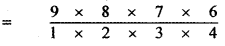= 9 × 2 × 7
= 126
Number of ways of choosing 2 persons (other than 2 children) from the remaining 5 persons (excluding children) = 5C2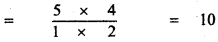Probability that exactly two of them are children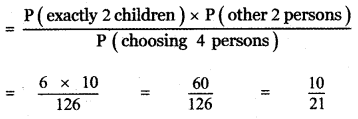Question 2.
A number is selected from the set (1, 2, 3, ….., 20}. The probability that the selected number is divisible by 3 or 4 is
(1) $$\frac{2}{5}$$
(2) $$\frac{1}{5}$$
(3) $$\frac{2}{5}$$
(4) $$\frac{2}{5}$$
(3) $$\frac{2}{5}$$

Explaination:
Sample space S is
S = {1, 2, 3, ………. , 20}
n(S) = 20
Let A be the event of selecting a number divisible by 3
A = {3, 6, 9, 12, 15, 18}
n(A) = 6
Let B be the event of selecting a number divisible by 4
B = {4, 8, 12, 16, 20}
n(B) = 5
A ∩ B = {12}
n(A ∩ B) = 1
P (Number divisible by 3 or 4) = P (A ∪ B)
= P(A) + P(B) – P(A ∩ B)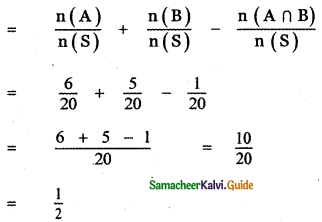Question 3.
A, B and C try to hit a target simultaneously but independently. Their respective probabilities of hitting the target are $$\frac{3}{4}$$, $$\frac{1}{2}$$, $$\frac{5}{8}$$. The probability that the target is hit by A or B but not by C is
(1) $$\frac{21}{64}$$
(2) $$\frac{7}{32}$$
(3) $$\frac{9}{64}$$
(4) $$\frac{7}{8}$$
(1) $$\frac{21}{64}$$

Explaination:
Given Probability of hitting the target by A is P(A) = $$\frac{3}{4}$$
Probability of hitting the target by B is P(B) = $$\frac{1}{2}$$
Probability of hitting the target by C is P(C) = $$\frac{5}{8}$$
Given A, B , C are Independent.
∴ P(A ∩ B ∩C) = P(A) P(B) . P(C)
Probability of the target hit by A or B but not by C is
P(A ∩ B ∩ C̅) = P(A ∪ B) . P(C̅)
= [P(A) + P(B) – P (A ∩ B)] [1 – P(C)]
= [P(A) + P(B) – P(A) P(B)] [1 – P(C)]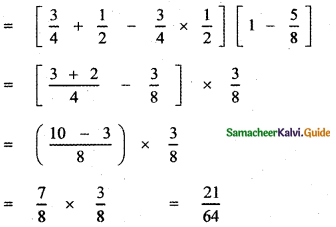Question 4.
If A and B are any two events, then the probability that exactly one of them occur is
(1 P(A ∪ B̅) + P(A̅ ∪ B)
(2) P(A ∩ B̅) + P(A̅ ∩ B)
(3) P(A) + P(B) – P(A ∩ B)
(4) P(A) + P(B) + 2P(A ∩ B)
(2) P(A ∩ B̅) + P(A̅ ∩ B)

Explaination:
Let A and B be an two events
The probability that exactly one of them occur is
= P(A ∩ B̅) + P(A̅ ∩ B)Question 5.
Let A and B be two events such that
P$$(\overline{\mathbf{A} \cup \mathbf{B}})$$ = $$\frac{1}{6}$$, P(A ∩ B) = $$\frac{1}{4}$$ and P(A̅) = $$\frac{1}{4}$$
Then the events A and B are
(1) Equally likely but not independent
(2) Independent but not equally likely
(3) Independent and equally likely
(4) Mutually inclusive and dependent
(2) Independent but not equally likely

Explaination: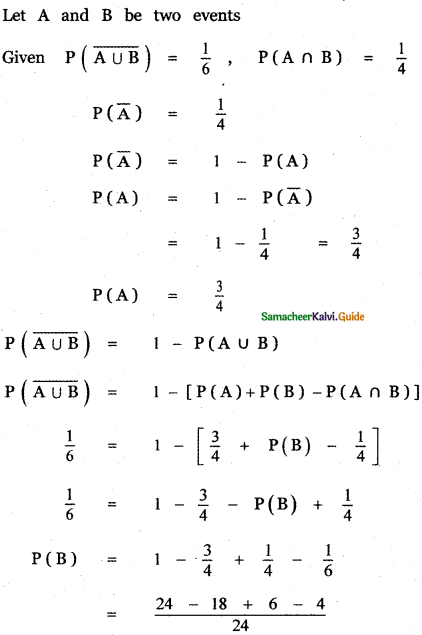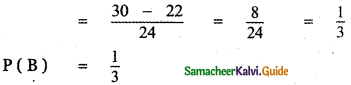If P(A ∩ B) = p(A) . p(B), then A and B are independent.
we have (A ∩ B) = $$\frac{1}{4}$$ ………… (1)
P(A) . P(B) = $$\frac{3}{4} \times \frac{1}{3}=\frac{1}{4}$$ ………… (2)
From equations (1) and (2) we get
P(A ∩ B) = p(A) . p(B)
∴ A and B are independent
Since P(A) ≠ P(B), not equally likely.
∴ A and B are independent but not equally likely.Question 6.
Two items are chosen from a lot containing twelve items of which four are defective, then the probability that at least one of the item is defective
(1) $$\frac{19}{33}$$
(2) $$\frac{17}{33}$$
(3) $$\frac{23}{33}$$
(4) $$\frac{13}{33}$$
(1) $$\frac{19}{33}$$

Explaination:
Total number of items = 12
Number of ways of choosing two items from 12 items is = 12C2
Number of defective items = 4
Number of non defective items = 8
Probability of getting atleast one defective items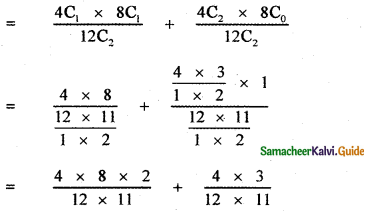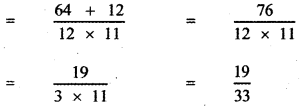Question 7.
A man has 3 fifty rupee notes, 4 hundred rupees notes and 6 five hundred rupees flotes in his pocket. If 2 notes are taken at random, what are the odds in favour of both notes being of hundred rupee denomination?
(1) 1 : 12
(2) 12 : 1
(3) 13 : 1
(4) 1 : 3
(1) 1 : 12

Explaination:
Let S be the sample space and A be the event of taking 2 hundred rupee notes.
n(S) = 13C2 = $$\frac{13 \times 12}{1 \times 2}$$ = 13 × 6
n(S) = 78
n(A) = 4C2 = $$\frac{4 \times 3}{1 \times 2}$$ = 2 × 3
n(A) = 6
n(A̅) = n(S) – n(A)
= 78 – 6 = 72
∴ Odds in favour of A is 6 : 72
That is 1 : 12Question 8.
A letter is taken at random from the letters of the word ‘ASSISTAN’T’ and another letter is taken at random from the letters of the word ‘STATISTICS’. The probability that the selected letters are the same is
(1) $$\frac{7}{45}$$
(2) $$\frac{17}{90}$$
(3) $$\frac{29}{90}$$
(4) $$\frac{19}{90}$$
(4) $$\frac{19}{90}$$

Explaination:
Given words ‘ASSISTANT’ ‘STATISTICS
Sample space S = {(A, S, S, I, S, T, A, N, T)
(S,T, A, T, I, S, T, I, C, S)}
n(S) = {(A, S), (A, T), (A, A), (A, T), (A, I), (A, S), (A, T), (A, I), (A, C), (A, S) }
n(S) = 9 × 10 = 90
Let A be the event of se}ecting equal letters.
A = {(A, A), (A, A), (S, S), (S, S), (S, S), (S, S), (S, S), (S, S), (S, S), (S, S), (S, S), (1, 1), (1, 1), (T, T), (T,T) , (T, T), (T, T), (T, T), (T, T) }
n(A) = 19
Probability of getting equal letters = $$\frac{19}{90}$$

Question 9.
A matrix is chosen at random from a set of all matrices of order 2, with elements 0 or 1 only. The probability that the determinant of the matrix chosen is non zero will be
(1) $$\frac{3}{16}$$
(2) $$\frac{3}{8}$$
(3) $$\frac{1}{4}$$
(4) $$\frac{5}{8}$$
(2) $$\frac{3}{8}$$

Explaination:
Sample space S = Set of all 2 × 2 matrices with elements 0 or 1 only
Number of elements in S is
n(S) = 24 = 16
Let A be the event of getting 2 × 2 matrices with elements 0 or 1 only whose determinant is non zero.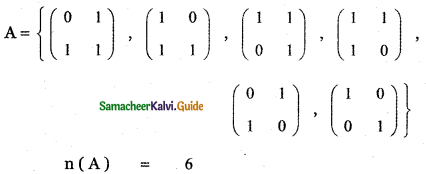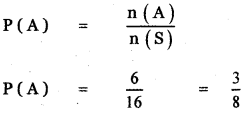Question 10.
A bag contains 5 white and 3 black balls. Five balls are drawn successively without replacement. The probability that they are alternately of different colours is
(1) $$\frac{3}{14}$$
(2) $$\frac{5}{14}$$
(3) $$\frac{1}{14}$$
(4) $$\frac{9}{14}$$
(3) $$\frac{1}{14}$$

Explaination:
Number of White balls (W) = 5
Number of Black balls (B) = 3
Five balls are drawn successively without replacement.
Probability that they are alternately of different
colours = P (W B W B W) + P (BW BW B)
= P(W) . P(B) . P(W) . P(B) . P(W) + P(B) . P(W) . P(B) . P(W) . P(B)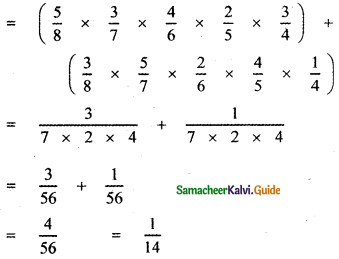Question 11.
If A and B are two events such that A ⊂ B and P(B) ≠ 0, then which of the following is correct?
(1) P(A/B) = $$\frac{\mathbf{P}(\mathbf{A})}{\mathbf{P}(\mathbf{B})}$$
(2) P(A/B) < P(A) (3) P(A/B) ≥ P(A) (4) P(A/B) >P(A)
(3) P(A/B) ≥ P(A)

Explaination:
Given A and B are two events such that A ⊆ B
and P(B) ≠ 0 then
P(A/B) ≥ P(A)Question 12.
A bag contains 6 green, 2 white , and 7 black balls. If two balls are drawn simultaneously, then the probability that both are different colours is
(1) $$\frac{68}{105}$$
(2) $$\frac{71}{105}$$
(3) $$\frac{64}{105}$$
(4) $$\frac{73}{105}$$
(1) $$\frac{68}{105}$$

Explaination:
Number of green balls (G) = 6
Number of white balls (W) = 2
Number of black balls (B) = 7
Two balls are drawn simultaneously
P (Balls are of different colours)
= P[(GW or WG) or (WB or BW) or (BG or GB)]
= P(GW) + P(WG) + P(WB) + P(BW) + P(BG) + P(GB)
= P(G) P(W) + P(W) P(G) + P(W) P(B) + P(B) P(W) + P(B) P(G) + P(G) P(B)
= 2 [P(G) P(W) + P(W) P(B) + P(B) P(G)]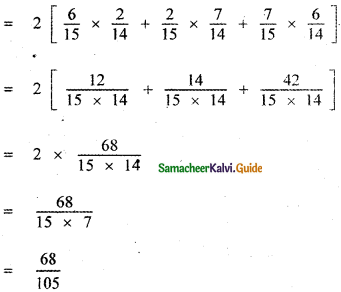Question 13.
If X and Y be two events such that
P(X/Y) = $$\frac{1}{2}$$, P(Y/X) = $$\frac{1}{3}$$ and P( X ∩ Y) = $$\frac{1}{6}$$,then
(1) $$\frac{1}{3}$$
(2) $$\frac{2}{5}$$
(3) $$\frac{1}{6}$$
(4) $$\frac{2}{3}$$
(4) $$\frac{2}{3}$$

Explaination: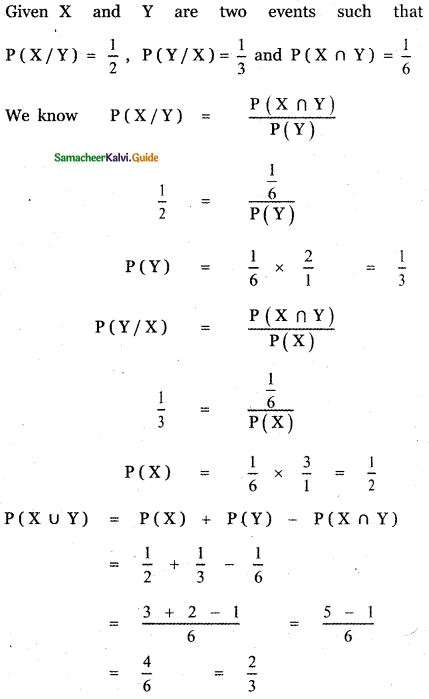Question 14.
An urn contains 5 red and 5 black balls. A ball is drawn at random, its colour is noted and is returned to the urn. Moreover, 2 additional balls of the colour drawn are put in the urn and then a ball is drawn at random. The probability that the second ball drawn is red will be
(1) $$\frac{5}{12}$$
(2) $$\frac{1}{2}$$
(3) $$\frac{5}{12}$$
(4) $$\frac{5}{12}$$
(2) $$\frac{1}{2}$$

Explaination:
Number of Red balls n (R) = 5
Number of Black halls n (B) = 5
Number of elements in the sample space n (S) = 5 + 5 = 10

Case (i)
P (drawing a red ball first) = $$\frac{5}{10}$$
Let P(E1) = $$\frac{1}{2}$$
Now two red balls are added.
P (drawing a red ball after adding) = $$\frac{7}{12}$$
P(A/E1) = $$\frac{7}{12}$$

Case (ii)
P (drawing a black ball first) = $$\frac{5}{10}$$
Let P(E2) = $$\frac{1}{2}$$
Now two black halls are added.
P (drawing a black ball after adding) = $$\frac{5}{12}$$
P(A/E2) = $$\frac{5}{12}$$
P(A) = P(A/E1) . P(E1) + P(A/E2) . P(E2)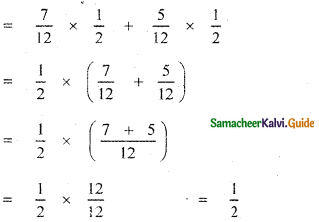Question 15.
A number x is chosen at random from the first 100 natural numbers. Let A be the event of numbers which satisfies $$\frac{(x-10)(x-50)}{x-30}$$ ≥ 0 then P(A) is
(1) 0.20
(2) 0.51
(3) 0.71
(4) 0.70
(3) 0.71

Explaination:
Given x is choosen from the first 100 natural numbers.
n(S) = 100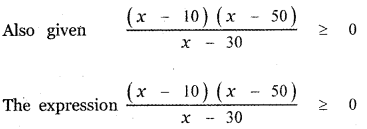Satifies when x takes the values 31 to 100 and also at x = 10
∴ A = { 10, 31, 32, 33, ……………, 100}
n(A) = 71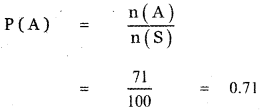Question 16.
If two events A and B are independent such that P(A) = 0.35 and P(A ∪ B) = 0.6, then P(B) is
(1) $$\frac{5}{13}$$
(2) $$\frac{1}{13}$$
(3) $$\frac{4}{13}$$
(4) $$\frac{7}{13}$$
(1) $$\frac{5}{13}$$

Explaination:
Given A and B are independent events
P(A ∩ B) = P(A) P(B)
Also given P(A) = 0.35 and P(A ∪ B) = 0.6
P(A ∪ B) = P(A) – P(B) – P(A ∩ B)
P(A ∪ B) = P(A) + P(B) – P(A) . P(B)
0.6 = 0.35 + P(B) – 0.35 P(B)
0.6 = 0.35 + (1 – 0.35) P (B)
0.6 = 0.35 + 0.65 P (B)
0.65 P (B) = 0.6 – 0.35
P(B) = $$\frac{0.25}{0.65}$$
P(B) = $$\frac{25}{65}$$ = $$\frac{5}{13}$$Question 17.
If two events A and B are such that P(A̅) = $$\frac{3}{10}$$ and P(A ∩ B̅) = $$\frac{1}{2}$$ then P(A ∩ B) is
(1) $$\frac{1}{2}$$
(2) $$\frac{1}{3}$$
(3) $$\frac{1}{4}$$
(4) $$\frac{1}{5}$$
(4) $$\frac{1}{5}$$

Explaination: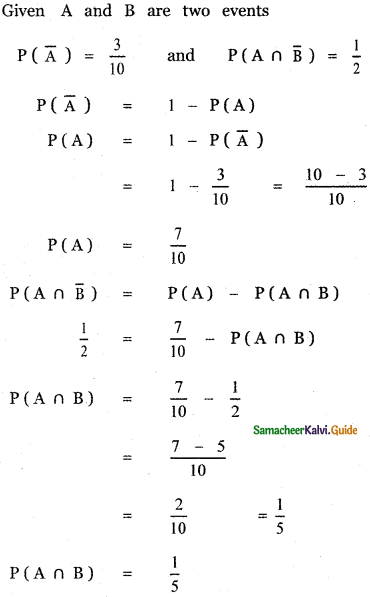Question 18.
If A and B are two events such that P(B) = and P(B/A) = 0.6,then P(A̅ ∩ B) is
(1) 0.96
(2) 0.24
(3) 0.56
(4) 0.66
(3) 0.56

Explaination:
Given A and B are two events.
P(A) = 0.4, P(B) = 0.8 and P(B/A) = 0.6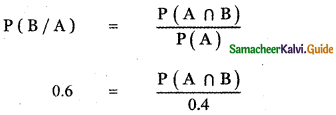P (A ∩ B) = 0.6 × 0.4 = 0.24
P(A̅ ∩ B) = P(B) – P(A ∩ B)
= 0.8 – 0.24
P(A̅ ∩ B) = 0.56Question 19.
There are three events A, B and C of which one and only one can happen. If the odds are 7 to 4 against A and 5 to 3 against B, then odds against C is
(1) 23 : 65
(2) 65 : 23
(3) 23 : 88
(4) 88 : 23
(2) 65 : 23

Explaination: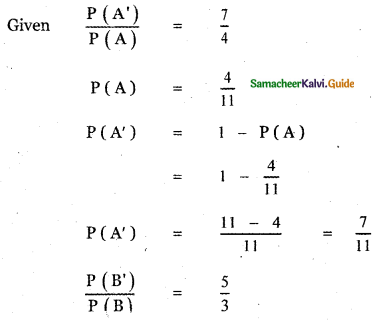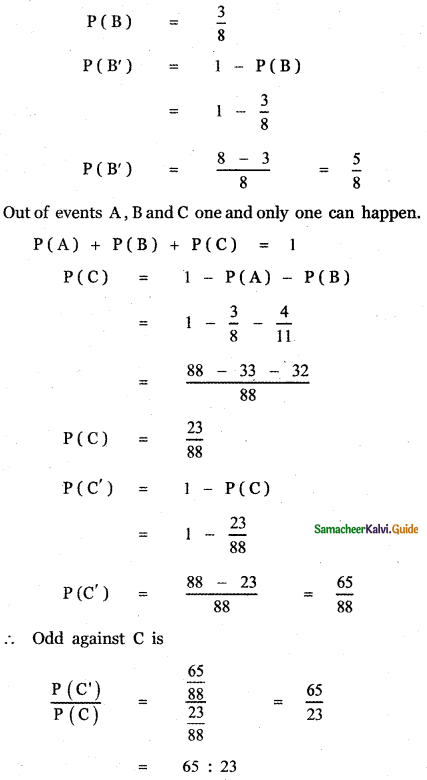Question 20.
If a and b are chosen randomly from the set {1, 2, 3, 4} with replacement, then the probability of the real roots of the equation x2 + ax + b = 0
(1) $$\frac{3}{16}$$
(2) $$\frac{3}{16}$$
(3) $$\frac{3}{16}$$
(4) $$\frac{3}{16}$$
(3) $$\frac{3}{16}$$

Explaination:
x2 + ax + b = 0 ⇒ x = $$\frac{-a \pm \sqrt{a^{2}-4 b}}{2}$$
Given that the roots are real ⇒ a2 – 4b ≥ 0 or a2 > 4b
When a = 1, b = 1 or 2 or 3 or 4 a2 – 4b < 0
When a = 2, b = 1 a2 – 4b = 0
When a = 3, b = 1 or 2 for which a2 – 4b ≥ 0
When a = 4, b = 1 or 2, 3 or 4 for which a2 – 4b ≥ 0
So, Selecting from the 4 number 42 = 16 ways.
(i.e.,) n(s) = 16
n(A) = (2 or 3 or 4) = 3
n(B) = (1 or 2 or 3 or 4) = 4
P(A) + P(B) = $$\frac{3}{16}+\frac{4}{16}=\frac{7}{16}$$

Question 21.
It is given that the events A and B are such that P(A) = $$\frac{1}{4}$$, P(A/B) = $$\frac{1}{2}$$ and P(B/A) = $$\frac{2}{3}$$. Then P(B) is
(1) $$\frac{1}{2}$$
(2) $$\frac{1}{2}$$
(3) $$\frac{1}{2}$$
(4) $$\frac{1}{2}$$
(2) $$\frac{1}{2}$$

Explaination:
Given A and B are two events.
Given P(A) = $$\frac{1}{4}$$, P(A/B) = $$\frac{1}{2}$$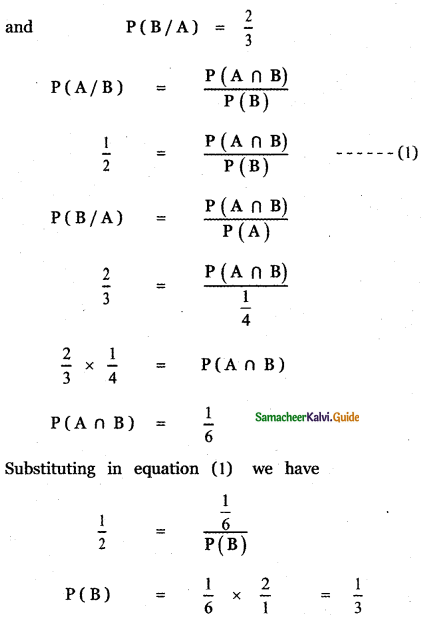Question 22.
In a certain college, 4 % of the boys and 1 % of the girls are taller than 1.8 meters. Further 60 % of the students are girls. If a student is selected at random and is taller than 1.8 meters, then the probability that the student is a girl is
(1) $$\frac{2}{11}$$
(2) $$\frac{3}{11}$$
(3) $$\frac{5}{11}$$
(4) $$\frac{7}{11}$$
(2) $$\frac{3}{11}$$

Explaination:
Let A1, A2, and B be the event of selecting a boy, selecting a girl, and selecting a tall student respectively.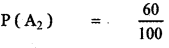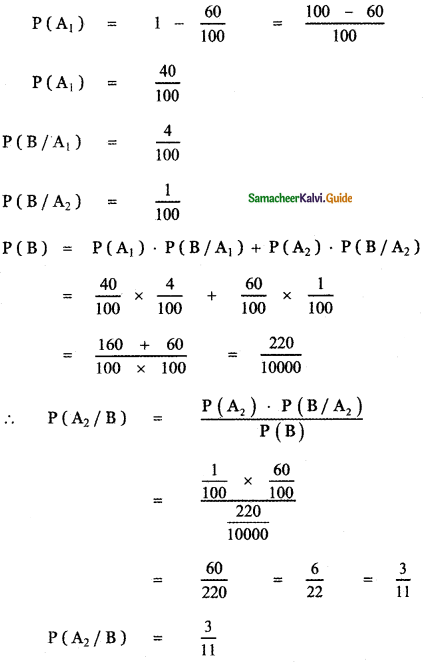Question 23.
Ten coins are tossed. The probability of getting at least 8 heads is
(1) $$\frac{7}{64}$$
(2) $$\frac{7}{32}$$
(3) $$\frac{7}{16}$$
(4) $$\frac{7}{128}$$
(4) $$\frac{7}{128}$$

Explaination:
Favourable events for atleast 8 heads
n(A) = 10C8 + 10C9 + 10C10
= 10C2 + 10C1 + 10C0
= $$\frac{10 \times 9}{1 \times 2}$$ + 10 + 1
= 5 × 9 + 11 = 45 + 11
n(A) = 56
Ten coins are tossed
∴ n(S) = 210 = 1024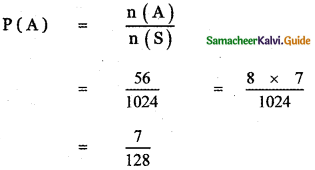Question 24.
The probability of two events A and B are 0.3 and 0.6 respectively. The probability that both A and B occur simultaneously is 0.18. The probability that neither A nor B occurs is
(1) 0.1
(2) 0.72
(3) 0.42
(4) 0.28
(4) 0.28

Explaination:
P(A) = 0.3, P(B) = 0.6
P(A ∩ B) = 0.18
So P(A ∪ B) = P(A) + P(B) – P(A ∩ B)
= 0.3 + 0.6 – 0.18
= 0.9 – 0.18 = 0.72
P(A’ ∩ B’) = P[(A ∪ B)’] = 1 – P(A ∪B)
= 1 – 0.72 = 0.28

Question 25.
If m is a number such that m ≤ 5, then the probability that quadratic equation 2x2 + 2mx + m + 1 = 0 has real roots is
(1) $$\frac{1}{5}$$
(2) $$\frac{2}{5}$$
(3) $$\frac{3}{5}$$
(4) $$\frac{4}{5}$$
(3) $$\frac{3}{5}$$Explaination:
2x2 + 2mx + m + 1 = 0roots are real ⇒ m2 – 2m – 2 ≥ 0
Here m ≤ 5 ⇒ n(S) = 5
When m= 1,m2 – 2m – 2
When m = 2, m2 – 2m- 2
When m = 3, m2 – 2m – 2
When m = 4, m2 – 2n- 2
When m = 5, m2 – 2m – 2
⇒ n{A) = 3 and so P(A) = $$\frac{3}{5}$$